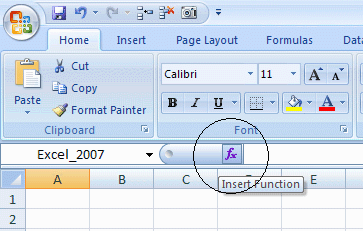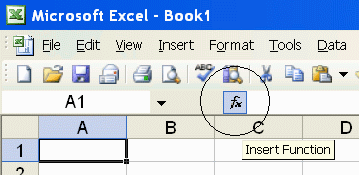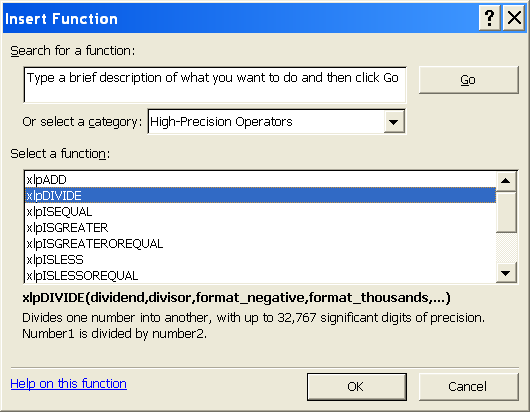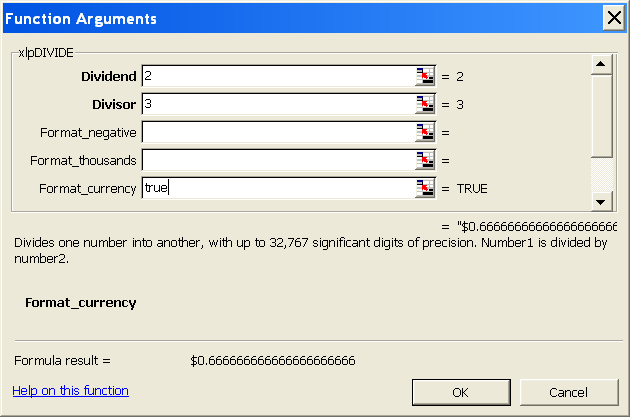PrecisionCalc
xl
Precision
Get Your Numbers Right

xlpMMULT

Returns the matrix product of two arrays, with up to 32,767 significant digits of precision. Same as Excel's built-in MMULT function, but with high precision.

A matrix product is in the form of an array, with the same number of rows as the first input array and the same number of columns as the 2nd input array.

xlpMMULT must be array-entered. To array-enter an xlpMMULT formula, follow these steps:

1. Select the cells in which you want the xlpMMULT formula.
2. With the cells selected, type the formula in the first (top or left) of the selected cells.
3. With the cells still selected, press and hold down the CTRL and SHIFT keys.
4. With the cells still selected, and with the CTRL and SHIFT keys still held down, press Enter.
5. You should see the xlpMMULT results in the selected cells.

xlpMMULT was introduced in xlPrecision 2017. It is not included in versions 3.x.x and earlier.

Syntax

xlpMMULT(array_rows,array_cols,format_negative,format_thousands,format_currency,exponential_notation,
maximum_significant_digits,format_decimal_place)

 array_rows Required. The array of rows to be multiplied by the array of columns. The numbers in the array's cells can be numbers, or text formatted as numbers. Any number of significant digits can be accepted. array_cols Required. The array of columns to be multiplied by the array of rows. The numbers in the array's cells can be numbers, or text formatted as numbers. Any number of significant digits can be accepted. format_negative Optional. Determines whether negatives are formatted with a leading hyphen ("-"), or parentheses ("()"). Set to 1 to format negatives with a leading hyphen. Set to 2 for parentheses. 1 by default. You can also format negatives with a red font. format_thousands Optional. Determines whether thousands separators are included. Set to TRUE to include thousands separators. FALSE by default. format_currency Optional. Determines whether currency symbol is included. Set to TRUE to include currency symbol. The currency symbol will be added either to the beginning or to the end of the result, whichever is appropriate for the locale. FALSE by default. exponential_notation Optional. Determines whether result is formatted in exponential notation. Set to TRUE to format in exponential notation. FALSE by default. maximum_significant_digits Optional. Determines the maximum number of significant digits to be returned. Default is 100, or the user's custom maximum set in the About box, or the maximum number allowed by the edition of xlPrecision, whichever is less. format_decimal_place Optional. Determines whether to add or remove decimal places as required to return the exact specified number of decimal places.When format_decimal_place causes decimal places to be removed, the remaining decimals are rounded, exactly as Excel's number formatting does. Set format_decimal_place to the number of decimal places desired. For example, set to 3 to specify that the result should have exactly 3 decimal places, so that: "123.4" is formatted as "123.400" "12.3456" is formatted as "12.346" "9.9999" is formatted as "10.000"

Use the Insert Function Dialog to easily enter these arguments:
(available on most editions of xlPrecision, but not recommended with the Free Edition as it causes the Free Edition dialog to appear multiple times)Examples

Formula Description Result
=xlpMMULT(A1:C2,A4:B6)
(array-entered in A8:B9)

 A B C 1 1 2 3 2 4 5 6 3 4 7 10 5 8 11 6 9 12
Matrix product of arrays of rows in cells A1:B2 and columns in cells A4:B6:

Cell A8:  (1 x 7) + (2 x 8) + (3 x 9)

Cell B8:  (1 x 10) + (2 x 11) + (3 x 12)

Cell A9:  (4 x 7) + (5 x 8) + (6 x 9)

Cell B9:  (4 x 10) + (5 x 11) + (6 x 12)

Return area has:
• 2 rows because array_rows has 2 rows, and:
• 2 columns because array_cols has 2 columns.
 A B 8 50 68 9 122 167
=xlpMMULT(A1:C2,A4:D6)
(array-entered in A8:D9)

 A B C D 1 1 2 3 2 4 5 6 3 4 7 10 13 16 5 8 11 14 17 6 9 12 15 18
Matrix product of arrays of rows in cells A1:C2 and columns in cells A4:D6:

Cell A8:  (1 x 7) + (2 x 8) + (3 x 9)

Cell B8:  (1 x 10) + (2 x 11) + (3 x 12)

Cell C8:  (1 x 13) + (2 x 14) + (3 x 15)

Cell D8:  (1 x 16) + (2 x 17) + (3 x 18)

Cell A9:  (4 x 7) + (5 x 8) + (6 x 9)

Cell B9:  (4 x 10) + (5 x 11) + (6 x 12)

Cell C9:  (4 x 13) + (5 x 14) + (6 x 15)

Cell D9:  (4 x 16) + (5 x 17) + (6 x 18)

Return area has:
• 2 rows because array_rows has 2 rows, and:
• 4 columns because array_cols has 4 columns.
 A B C D 8 50 68 86 104 9 122 167 212 257
=xlpMMULT(A1:C4,A6:B9)
(array-entered in A11:B14)

 A B C 1 1 2 3 2 4 5 6 3 7 8 9 4 10 11 12 5 6 13 16 7 14 17 9 15 18
Matrix product of arrays of rows in cells A1:C4 and columns in cells A6:B9:

Cell A11:  (1 x 13) + (2 x 14) + (3 x 15)

Cell B11:  (1 x 16) + (2 x 17) + (3 x 18)

Cell A12:  (4 x 13) + (5 x 14) + (6 x 15)

Cell B12:  (4 x 16) + (5 x 17) + (6 x 18)

Cell A13:  (7 x 13) + (8 x 14) + (9 x 15)

Cell B13:  (7 x 16) + (8 x 17) + (9 x 18)

Cell A14:  (10 x 13) + (11 x 14) + (12 x 15)

Cell B14:  (10 x 16) + (11 x 17) + (12 x 18)

Return area has:
• 4 rows because array_rows has 4 rows, and:
• 2 columns because array_cols has 2 columns.
 A B 11 86 104 12 212 257 13 338 410 14 464 563
=xlpMMULT(A1:C2,A4:B6)
(array-entered in A8:B9)

 A B C 1 111111111.1 2 3 2 4 5 6 3 4 777777777.7 10 5 8 11 6 9 12
Matrix product of arrays of rows in cells A1:B2 and columns in cells A4:B6:

Cell A8:
(111111111.1 x 777777777.7)
+ (2 x 8) + (3 x 9)

Cell B8:
(111111111.1 x 10) + (2 x 11) + (3 x 12)

Cell A9:
(4 x 777777777.7) + (5 x 8) + (6 x 9)

Cell B9:
(4 x 10) + (5 x 11) + (6 x 12)

Cell A8 has 19 significant digits. Excel's built-in MMULT function returns 86419753069135900 (not even rounded correctly).

 A B 8 8641975306 9135845.47 11111 11169 9 311111 1204.8 167
=xlpMMULT(A1:C2,A4:B6,2)
(array-entered in A8:B9)

 A B C 1 -1 -2 -3 2 -4 -5 -6 3 4 7 10 5 8 11 6 9 12
Negative numbers formatted with parentheses.

 A B 8 (50) (68) 9 (122) (167)
=xlpMMULT(A1:C2,A4:B6,,TRUE)
(array-entered in A8:B9)

 A B C 1 111111111.1 2 3 2 4 5 6 3 4 777777777.7 10 5 8 11 6 9 12
Formatted with localized thousands separators.

Decimal separators are also localized.

In the USA:

 A B 8 86,419, 753,069, 135,845.47 1,111, 111,169 9 3,111, 111,204.8 167

In Germany:

 A B 8 86.419. 753.069. 135.845,47 1.111. 111.169 9 3.111. 111.204,8 167

In France:

 A B 8 86 419 753 069, 135 845,47 1 111 111 169 9 3 111 111 204,8 167
=xlpMMULT(A1:C2,A4:B6,,,TRUE)
(array-entered in A8:B9)

 A B C 1 1 2 3 2 4 5 6 3 4 7 10 5 8 11 6 9 12
Formatted with the local currency symbol.

In the USA:

 A B 8 \$50 \$68 9 \$122 \$167

In Germany:

 A B 8 50 € 68 € 9 122 € 167 €

In Estonia:

 A B 8 50 kr 68 kr 9 122 kr 167 kr

In Albania:

 A B 8 50Lek 68Lek 9 122Lek 167Lek
=xlpMMULT(A1:C2,A4:B6,,,,TRUE)
(array-entered in A8:B9)

 A B C 1 11 22 33 2 44 55 66 3 4 7 10 5 8 11 6 9 12
Formatted in exponential notation.

 A B 8 5.5E+2 7.48E+2 9 1.342E+3 1.837E+3
=xlpMMULT(A1:C2,A4:B6,,,,,500)
=xlpMMULT(A1:C2,A4:B6,,,,,500000)
=xlpMMULT(A1:C2,A4:B6,,,,,A3)
Each number in the array is returned with up to 500 (or up to 500,000, or up to the number in A3) significant digits of precision.
=xlpMMULT(A1:C2,A4:B6,,,,,,2)
(array-entered in A8:B9)

 A B C 1 1.234 2.345 3.456 2 4 5 6 3 4 7 10 5 8 11 6 9 12
Formatted to 2 decimal places.

In cell A8, 58.50 is rounded (to the nearest) from 58.502.

In cell B8, 79.61 is rounded (to the nearest) from 79.607.

In cells A9 & B9, 122.00 & 167.00 are padded with zeros to two decimal places.

 A B 8 58.50_ 79.61_ 9 122.00_ 167.00_

Remarks

• xlPrecision results are returned as text that look like numbers, not as values that Excel recognizes as numbers. This is because Excel would truncate the results to 15 significant digits if it recognized them as numbers.

• Some arguments are ignored in some editions of xlPrecison. See the Buy page for details.

• maximum_significant_digits is ignored if it is higher than the maximum significant digits allowed by the edition of xlPrecision.

• Use maximum_significant_digits to increase calculation speed where desired. The lower the number used, the faster the calculation.

• array_rows and array_cols can accept both numbers and text.

• array_rows and array_cols can accept text formatted with the local currency symbol and thousands separators, and negatives can be formatted with either a leading hyphen or parentheses. They also can accept numbers formatted in scientific notation.

• array_rows and array_cols can accept text up to 32,767 characters long, depending on the edition of xlPrecision.

• You can use the results of xlPrecision functions as the operands in other xlPrecision formulas without losing any precision, but using them as operands in Excel's arithmetic functions will truncate them to 15 significant digits.

• If the return value is so large that it has more than 32,767 characters to the left of the decimal, then xlPrecision is of course unable to return a correct value and instead returns "#VALUE!". To return that data successfully, set exponential_notation to TRUE to return the value in exponential notation.

• 32,767 digits to the left of the decimal is a vastly larger number than Excel can return without xlPrecision. Excel itself can only return or recognize a number with no more than 308 digits to the left of the decimal.

• Decimal symbols, thousands separators, and currency symbols are all localized. This means that an xlPrecision formula that returns "\$1,234,567.89" in the USA will return "1.234.567,89 €" in Germany, "1 234 567,89 €" in France, "1 234 567.89 kr" in Estonia, and "1.234.567,89Lek" in Albania.

• The 32,767 SD edition can only provide a maximum of 32,767 total characters, including all formatting characters such as decimal, leading hyphen or parentheses for negatives, and thousands separators. As a result, it can only return the maximum 32,767 significant digits when the result is an unformatted positive integer. This is due to Excel's limitation of 32,767 characters in a cell. In all cases, the 32,767 SD edition will give you as many significant digits as possible with the formatting you have chosen.

• Depending on how many significant digits the edition of xlPrecision provides, the result may be too long to conveniently view. You can view the full result by right-clicking the cell and choosing Format Cells | Alignment | Wrap Text, and widening the column to the width of the screen. An easy way to view the full result without changing column widths or wrapping text is to right-click the cell, choose Copy, and then paste into Notepad or a word processor.

See Also

xlpMDETERM